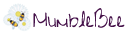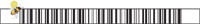# Free Amazon Android App of the Day 7/29/2014

Free Amazon Android App of the day for 7/29/2014 only!

Normally \$0.99 but for today it is FREE!!

# Math Formula Wizard

## Product Features

• Area calculator
• Volume calculator
• Distance Formula for points in a Cartesian coordinate system
• Binomial Theorem for exponents of 2 and 3
• Perimeter, Slope, Midpoint calculator
• Pythagoras calculator
• Custom calculator for any formula you can imagine
• Voice calculator

## Product Description

Math Formula Wizard – Math formulas and calculations on your Android phone or tablet. It’s free, beautiful and easy to use!

Formula Wizard is the perfect companion for anyone using mathematics in school, university, at work or for personal problems. The integrated calculator lets you calculate the area, volume and perimeter of any geometrical solid or shape. You can also use Formula Wizard to solve quadratic equations or to make use of the binomial theorem. On top of that, you can compute the distance between two points in a Cartesian coordinate system.

Get Formula Wizard for mathematics now and make your life easier! Whether you use this formula utility for your homework, to assist you at work or to prepare for an examination, it’s intuitive user interface and the calculating feature will help you a lot.

Follow Me On Social MediaThis site uses Akismet to reduce spam. Learn how your comment data is processed.